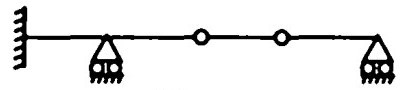Search

# Structural Analysis (1-20)

Updated: Apr 8, 2020

1. The number of independent equations to be satisfied for static equilibrium of a

plane structure is 3

2. If there are m unknown member forces, r unknown reaction components and j

number of joints, then the degree of static indeterminacy of a pin-jointed plane frame is given by (m + r - 2j)

3.Number of unknown internal forces in each member of a rigid jointed plane

frame is 3

4.Degree of static indeterminacy of a rigid jointed plane frame having 15 members, 3 reaction components and 14 joints is 6

5.Degree of kinematic indeterminacy of a pin-jointed plane frame is given by

2j - r

6.Independent displacement components at each joint of a rigid-jointed plane frame are-two linear movements and one rotation

7.If in a pin-jointed plane frame (m + r) >2j, then the frame is - stable and statically indeterminate

8.A pin-jointed plane frame is unstable if (m + r) < 2j

9.A rigid-jointed plane frame is stable and statically determinate if (3m + r) = 3j

10. Which of the beams shown in Fig. 12.1 is unstable ?11 Degree of kinematic indeterminacy of the beam shown in Fig. 12.2 is10

12. The rigid-jointed frame shown in Fig. 12.3 isstable and statically determinate

13.The degree of kinematic indeterminacy of the pin-joined frame shown in Fig. 12.4 is2

14. The number of independent equations to be satisfied for static equilibrium in a

space structure is 6

15. The degree of static indeterminacy of a pin-jointed space frame is given by

m + r - 3j

16. The degree of static indeterminacy of a rigid-jointed space frame is

6m + r - 6j

17. The degree of kinematic indeterminacy of a pin-jointed space frame is

3j-r

18. The number of independent displacement components at each joint of a rigid-jointed space frame is 6

19.If in a rigid-jointed space frame, (6m + r) < 6j, then the frame is -unstable

20.The principle of virtual work can be applied to elastic system by considering

the virtual work of-internal as well as external forces